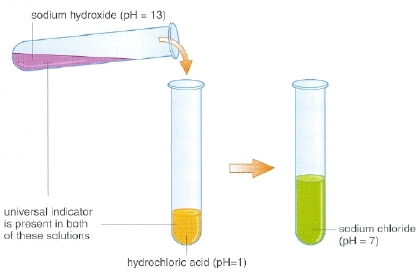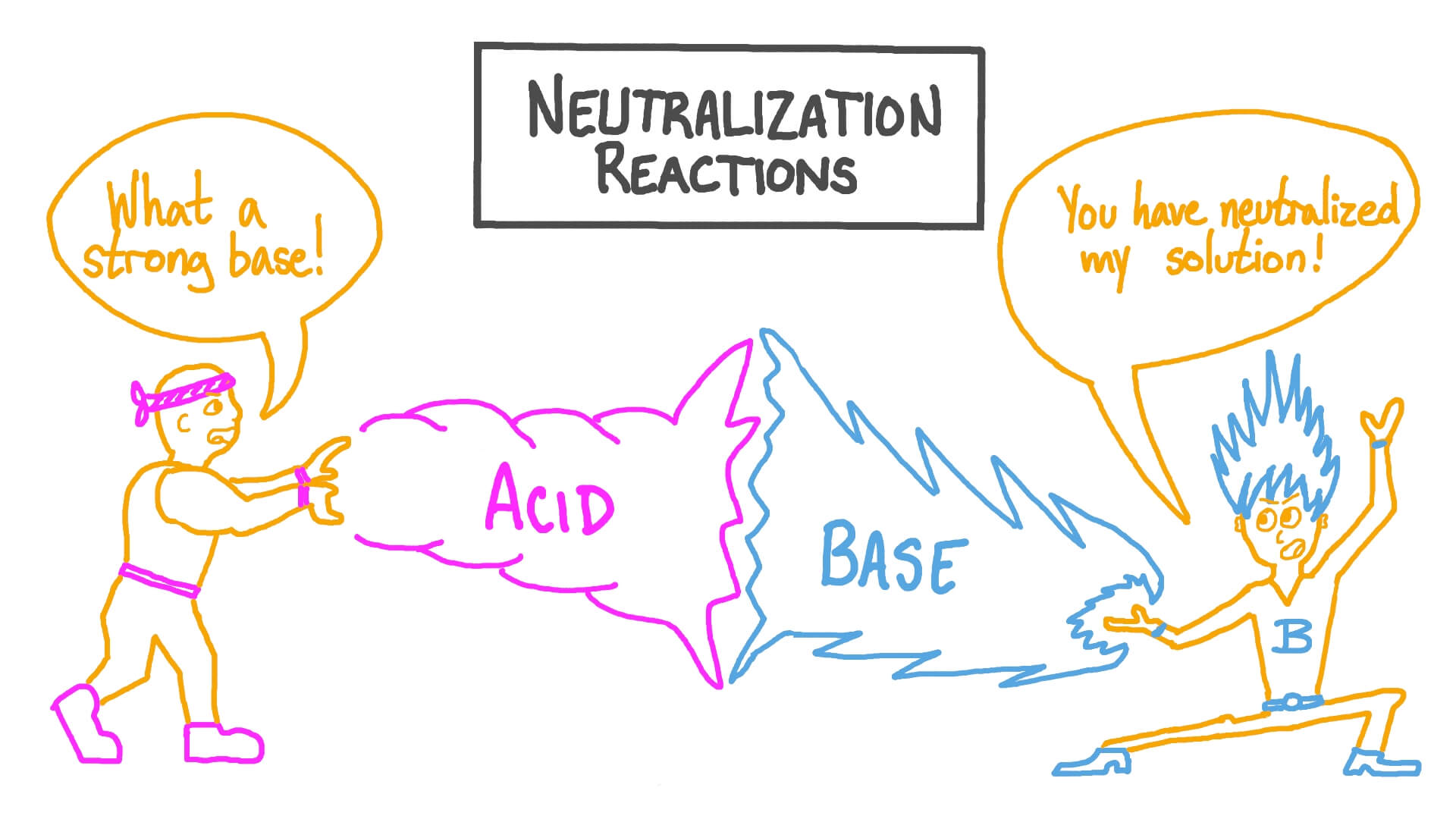# Experiment 4: Neutralization Reaction

Objective

Study reaction between acids and bases to understand neutralization

Materials required:

• Dilute HCL
• Dilute NaOH
• Phenolphthalein indicator
• Test tubes
• Dropper
• Test tube stand

Procedure:

• Take about 5 mL of dilute hydrochloric acid in a test tube
• Add 1-2 drops of phenolphthalein indicator to the solution and note down any change in colour
• Take about 10 mL of dilute sodium hydroxide solution in another test tube
• Take out sodium hydroxide solution with the help of a dropper and start adding this solution drop wise into the test tube containing hydrochloric acid till a change in colour is observed
• With the help of another dropper, take out hydrochloric acid and start adding it drop wise into the coloured solution obtained aboveFindings:

• Colour of the hydrochloric acid solution does not change on addition of phenolphthalein indicator
• On adding nearly 5 mL sodium hydroxide solution to the mixture of hydrochloric acid and phenolphthalein solution, the colour of the mixture changes to pink
• On addition of hydrochloric acid solution to the pink solution, the colour starts fading gradually and finally the solution becomes colourless

Conclusions:

• Phenolphthalein indicator remains colourless in the acidic solution while its colour changes to pink in basic solution
• It is found that on adding a base in an acid, a stage reaches when effect of acid is neutralised by base and vice-versa as indicated by colour change of indicator.

Theory:

• A neutralization reaction is when an acid and a base react to form water and a salt and involves the combination of H+ ions and OH- ions to generate water. The neutralization of a strong acid and strong base has a pH equal to 7
• When a solution is neutralized, it means that salts are formed from equal weights of acid and base. The amount of acid needed is the amount that would give one mole of protons (H+) and the amount of base needed is the amount that would give one mole of (OH-). Because salts are formed from neutralization reactions with equivalent concentrations of weights of acids and bases